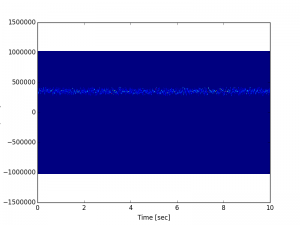http://web.stanford.edu/class/ee179/labs/Lab.html

http://aaronscher.com/

Seems hella useful

puts some data from my boy and puts it in file ab120_10.dat

rtl_sdr -f 120000000 -g 40 -n 20480000 ab120_10s.dat


-g sets gain. -f sets central frequency. Defaults to 2.048 samps/s. The n is number of samples which is 10x that so that means 10s.

I basically reimplemented the example code from matlab into  numpy.

A surprising glitch is that using pcolormesh with the fft/spectrogram is that you need to use the fftshift function for it to work right (hypothetically, it feels like this shouldn’t be necessary).

I was confused for a long time by this. The example code for spectrogram doesn’t use a complex signal so I figured that was the problem, but I could see the spike if I just plt.plot() a slice of time. This is bad behavior. I do not see this documented anywhere obvious.

rtl_sdr -f 101140000 -g 40 -n 20480000 ab120_10s.dat

from sys import argv
#This will allow a command line assignment of the filename
#script, filename = argv
import struct
import numpy as np
from scipy import signal
import matplotlib.pyplot as plt
import scipy as sp

filename = "ab120_10s.dat"

rawdata = np.fromfile(filename,dtype=np.uint8)
real = rawdata[0::2]-127.5
imag = rawdata[1::2]-127.5
vals = real + 1.j * imag
f, t, Sxx = signal.spectrogram(vals, 2048000,nperseg=512)

plt.pcolormesh(t[::100], np.fft.fftshift(f), np.fft.fftshift(Sxx[:,::100],axes=0))

plt.ylabel('Frequency [Hz]')
plt.xlabel('Time [sec]')
plt.show()Radio reference. Interesting listing of local radios. I don’t know how to decode p25. Some kind of digital radio standard

https://github.com/szechyjs/dsd

Maybe this is the right thing